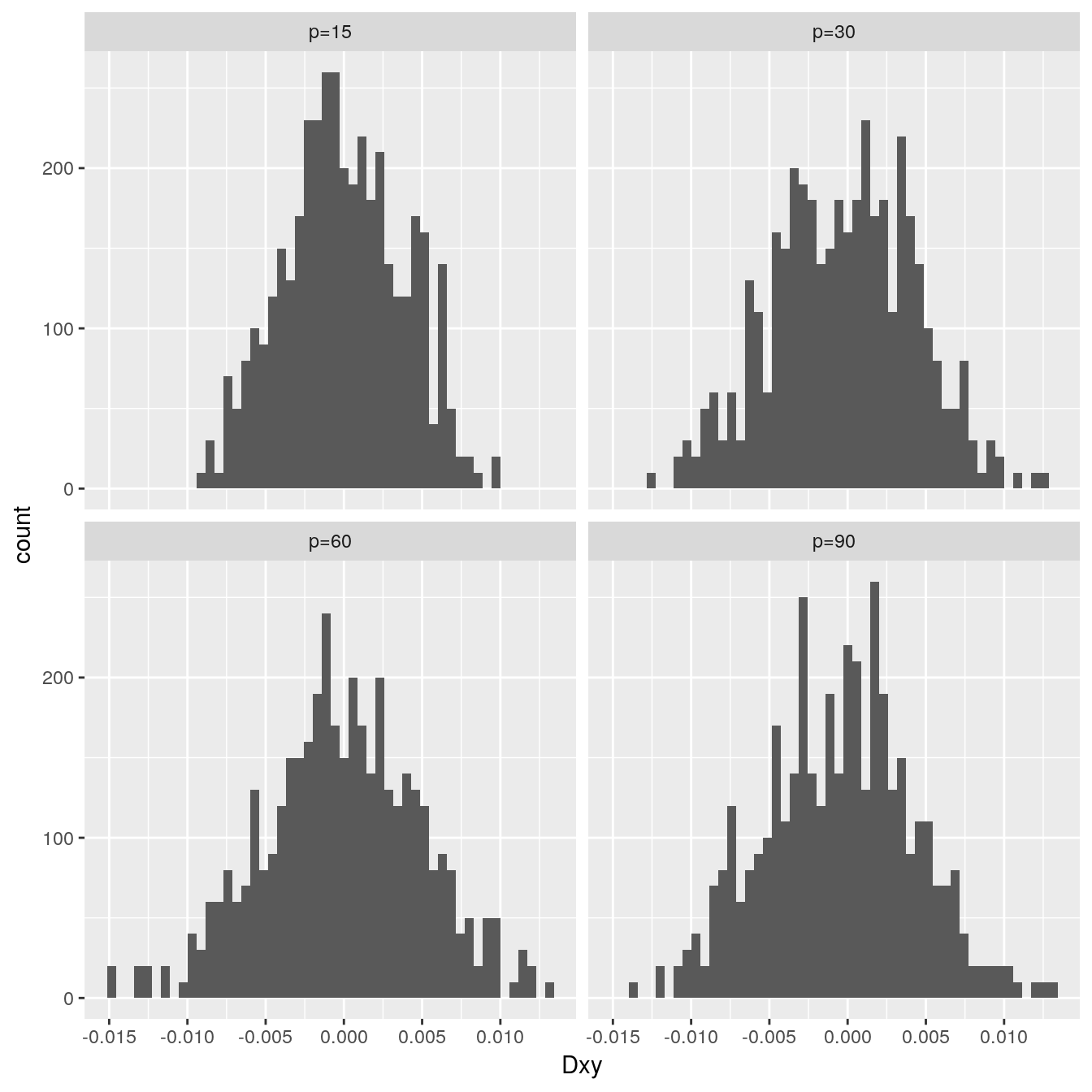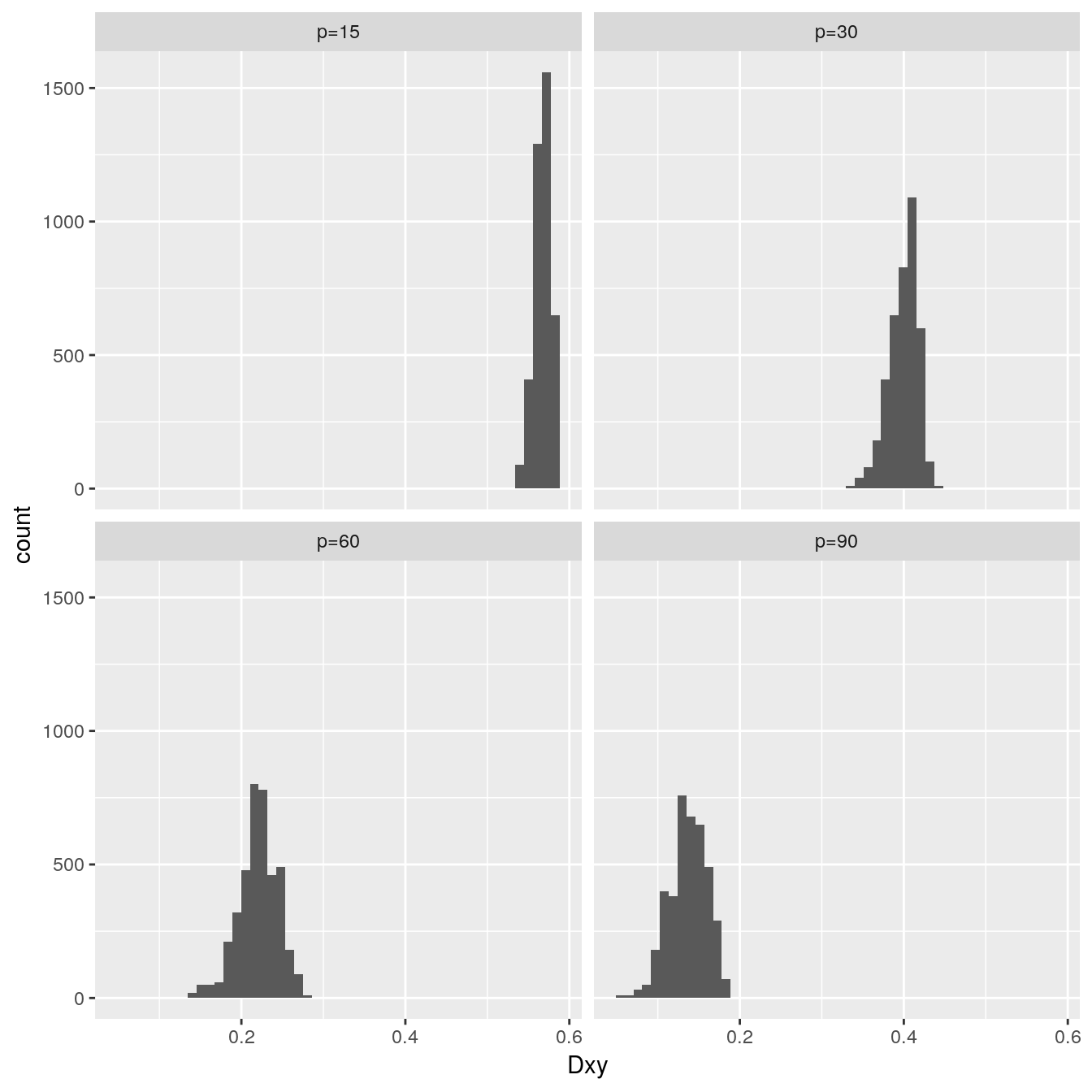# Simulation Method

For each of 400 simulations generate a training sample of 500 observations with p predictors (p=15, 30, 60, 90) and a binary reponse. The predictors are independently U(-0.5,0.5). The response is sampled so as to follow a logistic model where the intercept is zero and the regression coefficients have each of two patterns. First, all coefficients are set to 0.0 so that the true predictive model has no predictive discrimination ability (\(D_{xy}=0, c=\) AUROC \(=0.5\)). Then regression coefficients were uniformly spaced between -1 and 1, multiplied by a scaling factor that is < 1 when the number of predictors p is 30 and > 1 when p > 30. The “gold standard” is the predictive ability of the fitted model on a test sample containing 50,000 observations generated from the same population model. The task of a validation method is to recover this gold standard.

# Validation Methods

For each of the 400 training and validation samples, several validation methods were employed to estimate how the training sample model predicts responses in the 50,000 observations. These validation methods involve fitting 40 or 200 models per training sample.

g-fold cross-validation was done using the command `validate(f, method='cross', B=4 or B=10)` in the R `rms` package. This was repeated 4, 10, 20, or 50 times and averaged.

For bootstrap methods validate(f, method=‘boot’ or ‘632’, B=40 or B=200) was used. method=‘632’ does Efron’s “.632” method, labeled “632a” in the output. An ad-hoc modification of the .632 method, “632b” was also done. Here a “bias-corrected” index of accuracy is simply the index evaluated in the observation omitted from the bootstrap re-sample.

The “gold standard” external validations were done using the `val.prob` function in the `rms` package.

I didn’t run all combinations of validation methods and number of predictors, and I only used one sample size (500).

# Indexes of Predictive Accuracy

\(D_{xy}\): Somers’ rank correlation between predicted probability that Y=1 vs. the binary Y values. This equals 2(c - 0.5) where the c-index is the “ROC Area” or concordance probability.

Q: Logarithmic accuracy score - a scaled version of the log-likelihood achieved by the predictive model

Slope: calibration slope (slope of predicted log odds vs. true log odds)

# Measure of Accuracy of Validation Estimates

Three measures of accuracy of estimates of validated model performance are computed, in descending order of robustness and statistical efficiency.

• Root mean squared error of estimates (e.g., of \(D_{xy}\) from the bootstrap on the 500 observations) against the “gold standard” (e.g., \(D_{xy}\) for the fitted 500-observation model achieved in the 50,000 observations).
• Mean absolute error
• Median absolute error

# Code for the Simulations

``require(rms)``
``````options(prType='html')
knitrSet(lang='markdown')
mdchunk <- markupSpecs\$html\$mdchunk  # in Hmisc
dosims <- FALSE
set.seed(1)  # so can reproduce results
P <- c(15, 30, 60, 90)``````
``````n    <- 500         # Size of training sample
reps <- 400         # Simulations
npop <- 50000       # Size of validation gold standard sample
methods <- c('Boot 40','Boot 200','632a 40','632a 200',
'632b 40','632b 200','10-fold x 4','4-fold x 10',
'10-fold x 20','4-fold x 50')
R <- expand.grid(sim    = 1:reps,
p      = P,
method = methods,
sbeta  = 1:2)
R\$Dxy <- R\$Intercept <- R\$Slope <- R\$D <- R\$U <- R\$Q <- R\$repmeth <- R\$B <- NA
R\$n <- n
Rpop <- R

## Function to do r overall reps of B resamples, averaging to get
## estimates similar to as if r*B resamples were done

val <- function(fit, method, B, r) {
contains <- function(m) length(grep(m, method)) > 0
meth <- if(contains('Boot')) 'boot' else
if(contains('fold')) 'crossvalidation' else
if(contains('632')) '.632'
z <- 0
for(i in 1:r) z <- z + validate(fit, method=meth, B=B)[
c("Dxy","Intercept","Slope","D","U","Q"),'index.corrected']
z/r
}``````
``````for(sbeta in 1 : 2) {
for(p in P) {

## For each p create the true betas, the design matrix,
## and realizations of binary y in the gold standard large sample
Beta <- if(sbeta == 1) rep(0, p) else seq(-1, 1, length=p) * 30 / p
X    <- matrix(runif(npop*p), nrow=npop) - 0.5
LX   <- matxv(X, Beta)
Y    <- ifelse(runif(npop) <= plogis(LX), 1, 0)

## For each simulation create the data matrix and realizations of y
for(j in 1:reps) {
# cat(j, file='/tmp/z.txt')

## Make training sample
x <- matrix(runif(n*p), nrow=n) - 0.5
L <- matxv(x, Beta)
y <- ifelse(runif(n) <= plogis(L), 1, 0)
f <- lrm(y ~ x, x=TRUE, y=TRUE)
beta <- f\$coef
forecast <- matxv(X, beta)
## Validate in population
v <- val.prob(logit=forecast, y=Y, pl=FALSE)[
c("Dxy","Intercept","Slope","D","U","Q")]

for(method in methods) {
repmeth <- 1
if(method %in% c('Boot 40','632a 40','632b 40'))    B <- 40
if(method %in% c('Boot 200','632a 200','632b 200')) B <- 200
if(method == '10-fold x 4') {
B <- 10
repmeth <- 4
}
if(method == '4-fold x 10') {
B <- 4
repmeth <- 10
}
if(method == '10-fold x 20') {
B <- 10
repmeth <- 20
}
if(method == '4-fold x 50') {
B <- 4
repmeth <- 50
}

z <-  val(f, method, B, repmeth)
k <- which(R\$sim == j & R\$p == p & R\$method == method & R\$sbeta == sbeta)
if(length(k) != 1) stop('program logic error')
R[k, names(z)] <- z - v
R[k, c('B','repmeth','sbeta')] <- c(B=B, repmeth=repmeth, sbeta=sbeta)
Rpop[k, names(z)] <- v
Rpop[k, c('B', 'repmeth','sbeta')] <- c(B=B, repmeth=repmeth, sbeta=sbeta)
Save(R)  ## temporary until simulations are finished -
## can examine preliminary results
Save(Rpop)
} # end over methods
} # end over reps
} # end over p
} # end over sbeta

valSimresult <- R
Save(valSimresult)
valSimTrue <- Rpop
save(valSimTrue)``````

# Population Indexes

Let’s examine the “population” (true) values of \(D_{xy}\) that were achieved. For the null model simulations the true values are exactly zero.

## Distribution of Values for Null Models

A separate histogram is made for each number of predictors p.

``````Load(valSimresult)
v <- valSimTrue
v\$p <- paste0('p=', v\$p)
r <- subset(v, sbeta == 1)
require(ggplot2)
ggplot(r, aes(Dxy)) + facet_wrap(~ p) + geom_histogram(bins=50)``````## Distribution of Values for Non-Null Models

``````r <- subset(v, sbeta == 2)
ggplot(r, aes(Dxy)) + facet_wrap(~ p) + geom_histogram(bins=50)``````# Main Results

The three types of accuracies of the various estimators of forecast accuracy are summarized with multi-way dot charts. A trio of charts is produced first for the null models then for the non-null models.

``````Load(valSimresult)
dop <- function(sb) {
R <- subset(valSimresult, sbeta == sb)
statnames <- names(R)[7:12]
w <- reshape(R, direction='long', varying=list(statnames),
v.names='x', timevar='stat', times=statnames)
w\$p <- paste('p', w\$p, sep='=')

s <- with(w, summarize(x^2, llist(p, method, stat), smean.cl.boot,
stat.name='mse'))
P <- vector('list', 3)
P[] <-
ggplot(s, aes(x=sqrt(mse), y=method)) + geom_point() +
facet_grid(stat ~ p) +
geom_errorbarh(aes(xmin=sqrt(Lower), xmax=sqrt(Upper), y=method), height=0) +
xlab(expression(sqrt(MSE)))

s <- with(w, summarize(abs(x), llist(p, method, stat), smean.cl.boot,
stat.name='mae'))
P[] <-
ggplot(s, aes(x=mae, y=method)) + geom_point() +
facet_grid(stat ~ p) +
geom_errorbarh(aes(xmin=Lower, xmax=Upper, y=method), height=0) +
xlab('Mean |error|')

s <- with(w, summarize(abs(x), llist(p, method, stat), median,
stat.name='medae'))
P[] <-
ggplot(s, aes(x=medae, y=method)) + geom_point() +
facet_grid(stat ~ p) +
xlab('Median |error|')

mdchunk(robj=P, h=8, w=8,
cnames=paste0(c('rmse', 'mae', 'medae'), sb))
}``````

## Performance Under Null Models

``dop(1)``